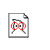# Evolutionary Algorithms for Learning Formulae in first-order Logic

Created by W.Langdon from gp-bibliography.bib Revision:1.5229

@PhdThesis{reiser:thesis,
• author = "Philip G. K. Reiser",
• title = "Evolutionary Algorithms for Learning Formulae in first-order Logic",
• school = "Computer Science, Aberystwyth, University of Wales",
• year = "1999",
• month = sep,
• keywords = "genetic algorithms, genetic programming, ILP",
• URL = "http://www.stancomb.co.uk/~prr/Papers/thesis.ps",
• URL = "http://www.stancomb.co.uk/~prr/Papers/thesis.pdf",
• size = "184 pages",
• abstract = "The successful application of machine learning algorithms depends on choosing the right space of hypotheses. Finding this space is a challenge that machine learning practitioners must regularly address. Ideally, one could specify a large hypothesis space and perform a complete search over it. However for most interesting problems this is intractable. Many algorithms make simplifying assumptions such as learning rules separately and combining them. Unfortunately, such approaches are prone to learning suboptimal hypotheses.

An alternative is stochastic search which may not necessarily return optimal hypotheses, but can provide a way of rapidly locating good ones and is more robust than greedy search strategies. This thesis concerns the use of evolutionary algorithms to perform stochastic search for formulae in (a subset of) first order logic. Evolution is viewed as a complex deductive-inductive process that conjectures new formulae and compares them against one another based on their logical consequences to determine their suitability as a solution.

Two algorithms were developed that comprised of two competing strategies: global reliability and local refinement. The first algorithm learns control strategies for discrete-time dynamical systems. Empirical studies revealed that although simple strategies could be learned, the evolutionary algorithm conjectured many formulae that are syntactically incorrect or semantically in valid. As a result, search was unnecessarily inefficient. By restricting consideration to the problem of classification (or concept learning) an explicit logical setting can be adopted that defines the valid formulae. The second algorithm, referred to as an `evolutionary wrapper', is a hybrid inductive logic programming (ILP) evolutionary algorithm. It can construct only classifiers that are consistent with the logical setting. Empirical investigations revealed that for some problems considerable increases in predictive accuracy can be achieved over ILP alone.",

• notes = "thesis.pdf does not work with xpdf but seems fine with acrobat",
}

Genetic Programming entries for Philip G K Reiser